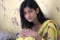## Finding the minimum and maximum value from a string with numbers separated by hyphen in MySQL?Sharon Christine

Updated on 30-Jul-2019 22:30:26

340 Views

Use MIN() function along with SUBSTRING() for minimum, whereas MAX() for maximum. Let us first create a table −mysql> create table DemoTable -> ( -> Id int NOT NULL AUTO_INCREMENT PRIMARY KEY, -> Value varchar(100) -> ); Query ... Read More

## Can we implement 'LIKE' and ‘IN’ in a single MySQL query?Sharon Christine

Updated on 30-Jul-2019 22:30:26

195 Views

For more efficiency, use Regular Expression for the same task. Let us first create a table −mysql> create table DemoTable -> ( -> Name varchar(30) -> ); Query OK, 0 rows affected (0.62 sec)Insert some records in the table using insert ... Read More

## C Program for Program to find the area of a circle?Sharon Christine

Updated on 30-Jul-2019 22:30:26

13K+ Views

The area is a quantity that represents the extent of the figure in two dimensions. The area of a circle is the area covered by the circle in a two dimensional plane.To find the area of a circle, the radius[r] or diameter[d](2* radius) is required.The formula used to calculate the ... Read More

## C Program to Add two IntegersSharon Christine

Updated on 30-Jul-2019 22:30:26

627 Views

A program to add two numbers takes to numbers and does their mathematical sum and gives it to another variable that stores its sum.Example Code Live Demo#include int main(void) {    int a = 545;    int b = 123;    printf("The first number is %d and the second number ... Read More

## Sum of first N natural numbers which are divisible by X or YSharon Christine

Updated on 30-Jul-2019 22:30:26

446 Views

Adding up all natural numbers up to n, that are divisible by X or Y is selecting all the numbers that are divisible by X or Y and adding them to a variable that stores the sum.To find the sum of the first N natural numbers which are divisible by ... Read More

## C++ vs Java vs Python?Sharon Christine

Updated on 30-Jul-2019 22:30:26

7K+ Views

Programs now has become an integrated part of our day to day life. Everything is automated now and connected with Iot.Amongst the programmers the most popular programming languages are c++ ; Java ; Python.C++ being a fast and compiled programming language has gained popularity and is the first programming language ... Read More

## Area of the largest triangle that can be inscribed within a rectangle?Sharon Christine

Updated on 30-Jul-2019 22:30:26

118 Views

A rectangle is a quadrilateral that has opposite side equal and parallel. The adjacent side are at 90o. And a triangle is a closed figure with three sides.The largest triangle inscribed within a rectangle. Has its base equal to the length of the rectangle and height of the triangle is ... Read More

## Power Function in C/C++Sharon Christine

Updated on 30-Jul-2019 22:30:26

582 Views

Power function is used to calculate the power of the given number.The pow function find the value of a raised to the power b i.e. ab.Syntaxdouble pow(double a , double b)It accepts a double integers as input and output a double integer as output. It pow() function is defined in ... Read More

## Average of Squares of Natural Numbers?Sharon Christine

Updated on 30-Jul-2019 22:30:26

2K+ Views

The average of the square of Natural Number is calculated by adding all the squares up to n natural numbers and then dividing it by the number.SampleAverage of square of first 2 natural numbers is 2.5 ,12 + 22 = 5 => 5/2 = 2.5.There are two methods for calculating ... Read More

## Area of circle inscribed within rhombus?Sharon Christine

Updated on 30-Jul-2019 22:30:26

2K+ Views

Circle inscribed in a rhombus touches its four side a four ends. The side of rhombus is a tangent to the circle.Here, r is the radius that is to be found using a and, the diagonals whose values are given.Now the area of triangle AOB = ½ * OA * ... Read More